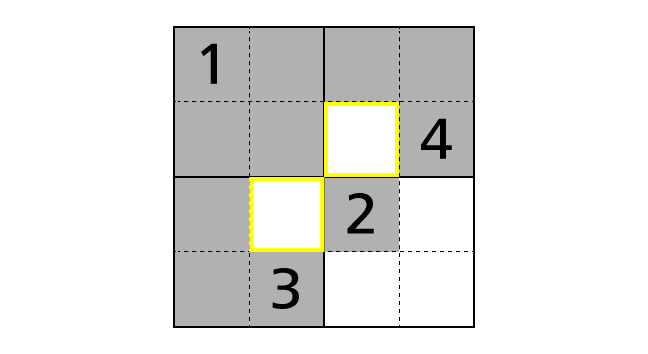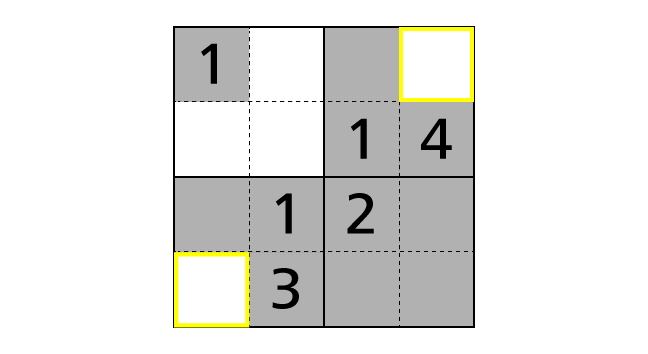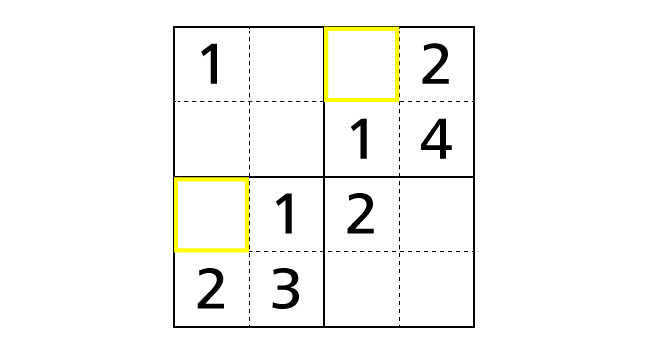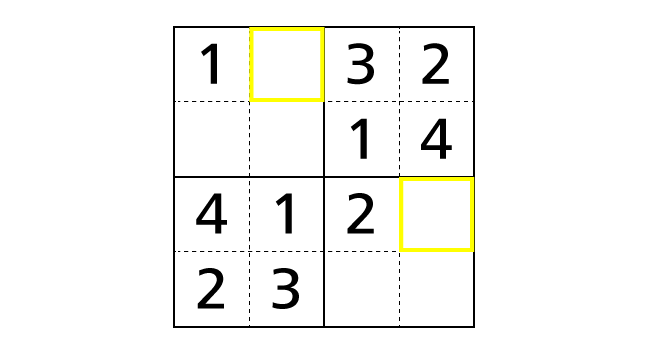# Rule and Tutorial of Sudoku

## Introduction

Sudoku 4×4 is a puzzle game which we have to determine the place of numbers by using given hints under some rules. Here we explain the rule of Sudoku 4×4 in detail for those who plays it for the first time. Then we demonstrate the solving process of an example puzzle.

In explanations, we call a horizontal sequence of boxes row and a vertical sequence of boxes column. We describe a position of a box as (1,1) using a row and a column number. For example, we call the colored box in the following image box–(2,3).## Rule

There are three rules for Sudoku 4×4. Please remember them all.

1. Each row contains each digit of 1–4 exactly once.
2. Each column contains each digit of 1–4 exactly once.
3. Each region surrounded by thick lines contains each digit of 1–4 exactly once.

Now we explain each rule in detail by using the example puzzle following.### Rule 1

Each row contains each digit of 1–4 exactly once. This means that we cannot place same numbers in a row. In the example, a number 1 is placed in box–(1,1). So we cannot place another number 1 in box–(1,2), box–(1,3) and box–(1,4).### Rule 2

Each column contains each digit of 1–4 exactly once. This means that we cannot place same numbers in a column. In the example, a number 1 is placed in box–(1,1). So we cannot place another number 1 in box–(2,1), box–(3,1) and box–(4,1).### Rule 3

Each region surrounded by thick lines contains each digit of 1–4 exactly once. This means that we cannot place same numbers in a region. In the example, we can see four regions. Box–(1,1), box–(1,2), box–(2,1) and box–(2,2) forms one regions. A number 1 is placed in box–(1,1). Then we cannot place another number 1 in box–(1,2), box–(2,1) and box–(2,2).## Tutorial

Here, we demonstrate a solving process of the example puzzle. This is not the only process to solve. We hope you will find other processes.

### Process 1

A basic technique to solve Sudoku 4×4 is to find boxes in which a specific number should be placed. For that, we focus on given hints. First, we focus on a number 1 of box–(1,1). We fill boxes in which we cannot place a number 1 with a color by following rules.This time, we notice that there is a box (surrounded by yellow lines) that number 1 is the only choice to be placed in. Because, by rule 3, we have to place a number 1 exactly once in the top right region. But, by rule 1, we cannot place a number 1 in box–(1,3) and box–(1,4). So box–(2,3) is the only box that we can place a number 1 in.
As the same way, in the bottom left region, box–(3,2) is the only box that we can place a number 1 in.

Now let’s place numbers.### Process 2

Next, we focus on number 2 in box–(3,3). Then we fill boxes in which we cannot place a number 2 with a color by following rules.We noticed that there are boxes that number 2 is the only choice to be placed in. Now let’s place numbers.### Process 3

Next, we focus on the top right region and the bottom left region. Then, by rule 3, we notice that box–(1,3) is the only box that we can place a number 3 in. Moreover, by rule 3, we notice that box–(3,1) is the only box that we can place a number 4 in.Now let’s place numbers.### Process 4

Next, we focus on row 1. Then, by rule 1, we know that each row contains each digit of 1–4 exactly once. But number 1, 2 and 3 are already placed in row 1. So box–(1,2) is the only box that we can place a number 4 in.
As the same way, by focusing on row 3, we notice that box–(3,4) is the only box that we can place a number 3 in.Now let’s place numbers.### Process 5

Next, we focus on column 1. Then, by rule 2, we know that each column contains each digit of 1–4 exactly once. But number 1, 2 and 4 are already placed in column 1. So box–(2,1) is the only box that we can place a number 3 in.
As the same way, by focusing on columns, we can place numbers in right boxes.